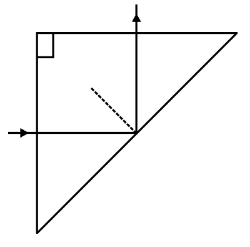(i) Plot a graph to show variations of the angle of deviation as a function

of angle of incidence for light passing through a prism. Derive an expression

for refractive index of the prism in terms of angle of minimum deviation

and angle of prism.

(ii) What is dispersion of light? What is its cause?

(iii) A ray of light incident normally on one face of a

right isosceles prism is totally reflected as shown in fig.

What must be the minimum value of refractive index

of glass? Give relevant calculations.                                 (5 marks)Explanation is a part of a Paid Course. To view Explanation Please buy the course.

Difficulty Level: